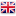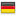# Sinergit Ltd.You are here:
27 September 2022

M1 = mass of the crane (kg)

M2 = mass of the trolley (kg) excluding weight of the load when suspended ropes

V = the very high speed of the crane (m / s)

MA = mass borne on the support A (kg)

MA = M1 / 2 + M2 * b / a

### the kinetic energy value on the support over loaded

And (support A) = MA * Vr ^ 2 / 2 0.7 where Vr = V * (if any of the electrical systems slowdown)### bumpers choice for two bridge cranes with opposing contemporary approach

MA1 = mass borne on the support A1 (kg) of 1 cranes

V1 = speed of 1 crane (m / s)

MA2 = mass borne on the support A2, as opposed to A1, 2 of the crane (kg)

V2 = speed of 2 crane (m / s)

KINETIC ENERGY VALUE on the support MOST LOADED

And (A1-A2 supports) = MA1 MA2 * * (+ V1r V2r) ^ 2 / 2 * (+ MA1 MA2)

where V1r = 0.7 * V1 (if any slowing of electrical systems)
where V2r = 0.7 * V2 (if any slowing of electrical systems)